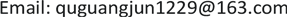﻿ 集值映射的Krasnoselskii型不动点 On the Krasnoselskii-Type Fixed Point of Set-Valued Mapping

Pure Mathematics
Vol.08 No.04(2018), Article ID:25971,5 pages
10.12677/PM.2018.84056

On the Krasnoselskii-Type Fixed Point of Set-Valued Mapping

Guangjun Qu

School of Mathematics and Computer Science, Shaanxi University of Technology, Hanzhong ShaanxiReceived: Jun. 27th, 2018; accepted: Jul. 12th, 2018; published: Jul. 19th, 2018ABSTRACT

Using E. Zeidler fixed point theorem, the fixed point of the operator A + B has been obtained, where A is from a single value mapping to a set-valued mapping.

Keywords:Set-Valued Mapping, Fixed Point1. 引言

Krasnoselskii不动点定理：

1) A连续且是紧的；

2) B是压缩映射；

3) $\forall x,y\in M,Ax+By\in M$

2. 预备知识

$graph\left(F\right)=\left\{\left(x,y\right)\in X×Y:y\in F\left(x\right)\right\}$

3. 主要结果及其证明

(f1) $F\left(M\right)$ 是一相对紧集；

(f2) F在M是上半连续的；

(f3) 集合 $F\left(x\right)$ 是非空闭凸集。

(i) $A\left(M\right)$ 是一个相对紧集；

(ii) A在M上是上半连续的；

(iii) B是一个压缩映射，且 $Ax+Bx\in M$$\forall x\in M$

(iv) 集合 $A\left(x\right)$ 是非空闭集，且 $\forall y\in M$$\left\{x\in M:\left(I-B\right)x\in Ay\right\}$ 是一个凸集。

$\left(I-B\right){x}_{0}=y$ ，所以 $I-B$ 是可逆的。

${\left(I-B\right)}^{-1}{x}_{n}={y}_{n}$${\left(I-B\right)}^{-1}x=y$ ，即证明 ${y}_{n}\to y$

$\begin{array}{c}‖{x}_{n}-x‖=‖\left(I-B\right){y}_{n}-\left(I-B\right)y‖\ge ‖{y}_{n}-y‖-‖B{y}_{n}-By‖\\ \ge ‖{y}_{n}-y‖-k‖{y}_{n}-y‖=\left(1-k\right)‖{y}_{n}-y‖\end{array}$

(a) $A\left(M\right)\subset \left(I-B\right)\left(M\right)$

(b) $A\left(M\right)$ 包含在M的一个紧子集里面；

(c) 如果 $\left(I-B\right){x}_{n}\to y$ ，那么存在 $\left\{{x}_{n}\right\}$ 的一个收敛子列 $\left\{{x}_{{n}_{k}}\right\}$

(d) $\forall y\in M,\left\{x\in M:\left(I-B\right)x\in Ay\right\}$ 是一个凸集。

${x}_{n}={\left(I-B\right)}^{-1}{y}_{n}$${x}_{0}={\left(I-B\right)}^{-1}{y}_{0}$ ，则有 $\left(I-B\right){x}_{n}\to \left(I-B\right){x}_{0}$

$I-B$ 不是可逆的。

$F\left(x\right)$ 是凸集(由(d)可得)。

② 验证F是图闭的。

$\left(I-B\right){y}_{0}={{x}^{\prime }}_{0}$$\left(I-B\right){y}_{0}={{x}^{\prime }}_{0}\in A\left({x}_{0}\right)$

${y}_{0}\in {\left(I-B\right)}^{-1}A{x}_{0}$ (因为A是闭值的)，所以F在M上是图闭的。

③ 验证 $F\left(x\right)$ 是闭值的。

④ 验证 $F\left(M\right)$ 是相对紧集。

$\left\{{y}_{n}\right\}$ ，存在 $\left\{{x}_{n}\right\}$ ，使得 ${y}_{n}\in {\left(I-B\right)}^{-1}A{x}_{n}$ ，那么一定存在 ${{x}^{\prime }}_{n}\in A{x}_{n}$ ，使得 $\left(I-B\right){y}_{n}={{x}^{\prime }}_{n}$ 。因为 $A\left(M\right)$ 包含在M的一个紧子集里面，所以存在收敛子列 $\left\{{{x}^{\prime }}_{{n}_{k}}\right\}$${{x}^{\prime }}_{{n}_{k}}\to u$ ，即存在 ${y}_{{n}_{k}}$ ，使得 $\left(I-B\right){y}_{{n}_{k}}={{x}^{\prime }}_{{n}_{k}}\to u$

⑤ 验证F是上半连续的。

$\left\{{y}_{n}\right\}\in {F}^{-1}\left(B\right)$ ，存在：

${x}_{n}\in F\left({y}_{n}\right)\cap B\subset F\left({y}_{n}\right)\subset F\left(M\right)$ ( $F\left(M\right)$ 是相对紧集)。

On the Krasnoselskii-Type Fixed Point of Set-Valued Mapping[J]. 理论数学, 2018, 08(04): 426-430. https://doi.org/10.12677/PM.2018.84056

1. 1. Liu, Y.C. and Li, Z.X. (2008) Kraasnolelskii Fixed Point Theorems and Applications. Proceedings of the American Mathematical Society, 136, 1213-1220. https://doi.org/10.1090/S0002-9939-07-09190-3

2. 2. Burton, T. (1998) A Fixed-Point Theorem of Kraasnolelskii. Applied Mathematics Letters, 11, 85-88. https://doi.org/10.1016/S0893-9659(97)00138-9

3. 3. Ok, E.A. (2009) Fixed Set Theorems of Kraasnolelskii Type. Proceedings of the American Mathematical Society, 137, 511-518. https://doi.org/10.1090/S0002-9939-08-09332-5

4. 4. Dhage, B.C. (2003) Remarks on Two Fixed-Point Theorems Involving the Sum and the Product of Two Operators. Computers & Mathematics with Applications, 46, 1779-1785. https://doi.org/10.1016/S0898-1221(03)90236-7

5. 5. Henry, D. (1981) Geometric Theory of Semilinear Parabolic Equations. Springer-Verlag, Berlin-New York. https://doi.org/10.1007/BFb0089647

6. 6. 俞建. 博弈论与非线性分析[M]. 北京: 科学出版社, 2007.